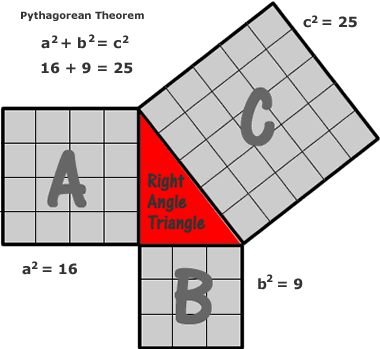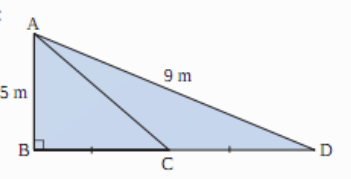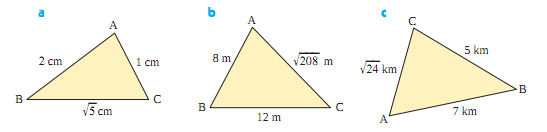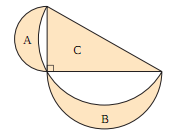The Learning Point‎ > ‎Mathematics‎ > ‎

Pythagoras Theorem:

4.1 Theorem:In any right triangle, the area of the square whose side is the hypotenuse (the side opposite the right angle) is equal to the sum of the areas of the squares whose sides are the two legs (the two sides that meet at a right angle). It is illustrated in the figure below:4.2.Proof:(Euclid's proof)
a) We label a few points (see the diagram), and draw lines BI and CE. ΔACE is congruent to ΔAIB, by the SAS theorem, because AC=AI, AE=AB and CAE=IAB (both angles are congruent to angle CAB + a right angle).
b) But, the area of ΔAIB is equal to half the area of square ACHI (They have the same base AI and height IH).
c) The area of triangle ACE is equal to half the area of the rectangle AGFE. So, the square ACHI is equal, in area, to the rectangle AGFE.
d) Likewise, we show that the area of the smaller square is equal to the area of the smaller rectangle.So, the area of the large square is equal to the sums of the areas of the two smaller squares.
e) Hence proved the pythagoras theorem.

4.3 Converse of pythagoras theorem: If in a triangle, square of one side is equal to the sum of the squares of the other two sides, then the angle opposite the first side is a right angle.

Visualizing the Pythagoras Theorem

4.4 Problems:

Q1. Jake leaves school to go home. He walks 6 blocks North and then 8 blocks west. How far is Jake from the school?

Answer: (62 + 82)0.5 = 1000.5 = 10 blocks away

Q2.Find the value of AC, Given that C is the midpoint of BD.Q3. Which of the following triangles a,b,c is a right angled triangles?Answer: All are right triangled. In each of them, the square of the largest side equals the sum of the squares of the other two sides.

Q4. In the figure below show that Area A + Area B = Area C.Pythagorean triple

Pythagorean triple consists of three positive integers ab, and c, such that a2 + b2 = c2. Such a triple is commonly written (abc)
Some Pythagorean triples are:

(3, 4, 5)
(5, 12, 13)
(8, 15, 17)
(7, 24, 25)
(20, 21, 29)
(12, 35, 37)
(9, 40, 41)
(28, 45, 53)
(11, 60, 61)
(16, 63, 65)
(33, 56, 65)
(48, 55, 73)
(13, 84, 85)
(36, 77, 85)
(39, 80, 89)
(65, 72, 97)